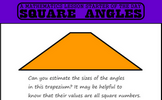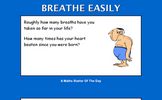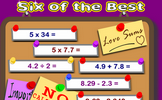# Developing geometric reasoning

### Term 3 starting in week 4 :: Estimated time: 3 weeks

• Understand and use the sum of angles at a point
• Understand and use the sum of angles on a straight line
• Understand and use the equality of vertically opposite angles
• Know and apply the sum of angles in a triangle
• Know and apply the sum of angles in a quadrilateral
• Solve angle problems using properties of triangles and quadrilaterals
• Solve complex angle problems

For higher-attaining pupils:

• Find and use the angle sum of any polygon
• Investigate angles in parallel lines
• Understand and use parallel line angle rules
• Use known facts to obtain simple proofs

This page should remember your ticks from one visit to the next for a period of time. It does this by using Local Storage so the information is saved only on the computer you are working on right now.

## Lesson Starters

Here are some suggestions for whole-class, projectable resources which can be used at the beginnings of each lesson in this block.

### 1st Lesson#### Hexagon

On a full page in the back of your exercise book draw a perfectly regular hexagon.

### 2nd Lesson#### Pentagon

On a full page in the back of your exercise book draw a perfectly regular pentagon.

### 3rd Lesson#### Square Angles

Find a trapezium, a triangle and a quadrilateral where all of the angles are square numbers.

### 4th Lesson#### Air Traffic Control

Work out which aircraft are in danger of colliding from their positions and direction of travel. An exercise in understanding bearings.

### 5th Lesson#### Mental Maths Test

The starter is a PowerPoint file containing a twenty question mental arithmetic test. It will advance from one question to the next automatically.

### 6th Lesson### 7th Lesson#### Six of the Best

Six calculations to perform without a calculator.

### 8th Lesson#### Not Too Close

This activity requires eight students to sit non consecutively on a grid of chairs.

### 9th Lesson#### Step Perimeter

Is it possible to work out the perimeter of this shape if not all the side lengths are given?

Some of the Starters above are to reinforce concepts learnt, others are to introduce new ideas while others are on unrelated topics designed for retrieval practice or and opportunity to develop problem-solving skills.

White Rose ResourcesEnd of block assessments provide a quick progress check at the end of each block of learning to make sure students have understood the content covered. This Scheme of Learning was produced by White Rose Maths and is used here with permission granted on 30th June 2021.For All: